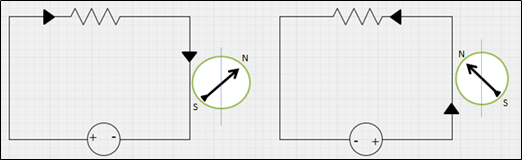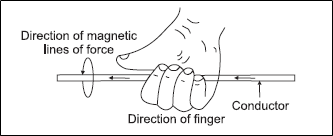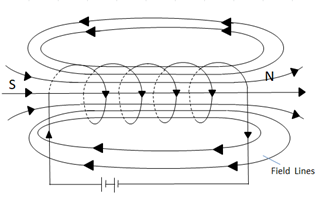## NCERT Class 10 Science : Learning Concept

### Science: Chapter 13-Magnetic Effects of Electric Current-Concepts

13.2 Magnetic Field Due to a Current Carrying conductor
To show this behavior, we use a circuit where current can be switched ON and OFF through a copper wire. A small compass is placed near the vicinity of the wire. When there is current flow through the wire, the compass needle will get deflected. Form this experiment we conclude that current flow inside a conductor creates a magnetic field which deflects the compass needle.Figure 2: Deflection of a compass needle near a current carrying conductor

One of the ways to determine the direction of magnetic field due to current flow in a conductor is the Right-Hand Thumb Rule.
Right-Hand Thumb Rule:
Imagine you are holding the conductor with the palm of your right hand, such that the fingers encircle the conductor and the thumb points in the direction of flow of current. Then the direction of the fingers encircling the conductor, gives the direction of the magnetic lines of force around it.Figure 3: Right Hand Thumb Rule

Field due to current carrying coil or solenoid:
In our experiment with a straight wire we don’t create a strong magnetic field with opposite poles instead we get a magnetic field visualized by concentric circles around the wire. With a solenoid we can create a field with recognizable poles.

What is a solenoid ?
A coil of many circular turns of insulated copper wire wrapped closely in the shape of a cylinder is called a solenoid.

Field lines inside a solenoid:Figure 4: Field Lines inside a solenoid
From the above picture of the field lines inside a solenoid, we found similarities of the field lines with the field lines bar magnet. Field lines leave the solenoid in the right side and enter the solenoid in the left side. The side where field lines leave the solenoid is considered as the north pole and the other side is considered as south pole. Inside the solenoid field lines are parallel to each other and thus the field is considered to be uniform.